Courses

# Columns Civil Engineering (CE) Notes | EduRev

## Civil Engineering (CE) : Columns Civil Engineering (CE) Notes | EduRev

The document Columns Civil Engineering (CE) Notes | EduRev is a part of the Civil Engineering (CE) Course RCC & Prestressed Concrete.
All you need of Civil Engineering (CE) at this link: Civil Engineering (CE)

Chapter 5

Columns
A. Working Stress Method 1. Slenderness ratio ( λ)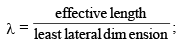; If λ ≤ 12 then the column is short, if λ > 12 then the column is long.

3. Load carying capacity for short column
P = σsc A sc + σcc . A c

A= Asc + Acc
Where, Ac = Area of concrete σsc  = Stress in compression steel
σcc = Stress in concrete

A = Total area Asc = Area of compression steel

4. Load carrying capacity for long column

P = Csc A sc + σcc . A c )
where,Cr =  Reduction factor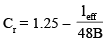or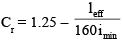where,leff = Effective length of column
B = Least lateral dimension
imin = Least radius of gryation and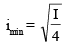where, I = Moment of inertia and A = Cross sectional area

5. Column with helical reinforcement
Strength of the column is increased by 5%
P = 1.05 (σsc A sc + σcc . A c) — For short column
P = 1.05Crsc A sc + σcc . A c ) – For  long column

Helical reinforcement is provided only for circular columns.

(i) Diameters of helical reinforcement is selected such that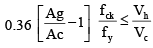(ii) Pitch of helical reinforcement: (p) (a) Fig. 39
where, dc = core diameter = dg – 2 × clear cover to helical reinforcement
Ag = gross area =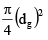dg = gross diameter

Vh = Volume of helical reinforcement in unit length of column
φh = diameter of steel bar forming the helix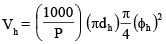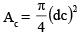V= Ac × 1

dh = centre of centre dia of helix = dg – 2 clear cover – φh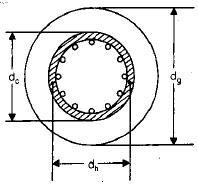6. Some other Indian Standards Recommendation 

• Longitudinal reinforcement
(a) Minimum area of steel = 0.8% of the gross area of column
(b) Maximum area of steel
(i) when bars are not lapped Amax = 6% of the gross area of column
(ii) when bars are lapped Amax = 4% of the gross area of column 
• Minimum number of bars for reinforcement For rectangular column — 4 For circular column — 6 
• Minimum diameter of bar = 12 mm 
• Maximum distance between longitudinal bar  = 300 mm 
• Pedestal : It is a short length whose effective length is not more than 3 times of least lateral dimension. In case of Pedestal minimum percentage of steel = 0.15%. 
• Transverse reinforcement (Ties)

φ = maximum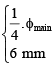where, φmain = dia of mainbar
φ = dia of bar for transverse reinforcement 

• Pitch (p)

φ = minimum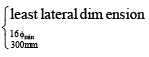where, φmin = minimum dia of bar

•  Slenderness limit
(i) Unsupported length between end restrains > 60  times least lateral dimension.
(ii) If in any given plane one end of column is unrestrained than its unsupported length >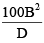 All column should be designed for a minimum eccentricity of emin = maximum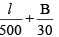20mm

B. Limit Stress Method

(1) Assumptions: All assumption for beams will be valid for column in addition to it there are two more assumptions.

(i) The maximum compressive strain in concrete in axial compression is take as 0.002

(ii) The maximum compresive stain at the highly compressed extreme fibre in concrete subjected to axial compression and bending and when there is no tension in the section shall be 0.0035 minus 0.75 times the strain at the least compressed extreme fibre.

(2) Minimum Eccentricity: All column should be designated for a minimum eccentricity of

emin = maximum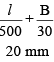(3) Design of Short Columns : When the minimum eccentricity does not exceed 0.05 B or 0.05 D then load carrying capacity of column is given by Pu = 0.4 fck Ac + 0.67 fy Asc
where, Pu = axial load on the column

(4) Short axially loaded column with helical reinforcement

Strength of the column is increased by 5% Pu = 1.05 (0.4 fck Ac + 0.67 fy Asc)

(5) Some others I.S Recommendation
(a) Slenderness limit

(i) Unsupported length between and restrains > 60 times least lateral dimension.

(ii) If in any given plane one end of column is unrestrained than its unsupported.
length >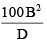where e = 0, i.e., the column is truly axially loaded.
Puz = 0.45 fck Ac + 0.75 fy Aac

Where Puz = Ultimate load carrying capacity of column This formula is also used for member subjected to combined axial load and bi-axial bending and also used when e > 0.05D.

Design of Long Columns

Long column is to be designed for moment + load if given values are
1.P
2. (Mux < Mu min) where Mu(min)  = Pue(min)]
3. (Mux < Mu min)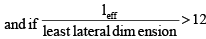Then, As per IS 456 : 2000
To consider the slenderness effect additional moments are added with given design moments.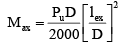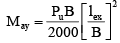Iex = effective length in respect of major axis.
Iey = effective length in respect of minor axis.
D = Depth of cross-section at right angle to the major axis.
B = Width of member

Final Design Values

1.  Pu
2. (Mux + Max)
3. (Muy + May)
The values Max and May may be multiplied by a factor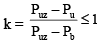where, Pv = axial load or compression member
Puz = ultimate load carrying capacity of  column
Pb = axial load corresponding to the condition of maximum compressive strain of 0.0035 in concrete and tensile of 0.002 in outer most layer of tension steel.

Offer running on EduRev: Apply code STAYHOME200 to get INR 200 off on our premium plan EduRev Infinity!

## RCC & Prestressed Concrete

14 videos|24 docs|12 tests

,

,

,

,

,

,

,

,

,

,

,

,

,

,

,

,

,

,

,

,

,

;